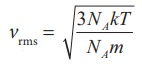Home | | Physics 11th std | Root mean square speed

# Root mean square speed

Root mean square speed (Vrms) is defined as the square root of the mean of the square of speeds of all molecules.

Root mean square speed (vrms)

Root mean square speed (vrms) is defined as the square root of the mean of the square of speeds of all molecules. It is denoted by vrms = √v2

Equation (9.8) can be re-written as,From the equation (9.18) we infer the following

(i) rms speed is directly proportional to square root of the temperature and inversely proportional to square root of mass of the molecule. At a given temperature the molecules of lighter mass move faster on an average than the molecules with heavier masses.

Example: Lighter molecules like hydrogen and helium have high ‘vrms’ than heavier molecules such as oxygen and nitrogen at the same temperature.

(ii) Increasing the temperature will increase the r.m.s speed of molecules

We can also write the vrms in terms of gas constant R. Equation (9.18) can be rewritten as followsSince NAk = R and NAm = M (molar mass)

The root mean square speed or r.m.s speedThe equation (9.6) can also be written in terms of rms speedImpact of vrms in nature:

### 1. Moon has no atmosphere.

The escape speed of gases on the surface of Moon is much less than the root mean square speeds of gases due to low gravity. Due to this all the gases escape from the surface of the Moon.

### 2. No hydrogen in Earth’s atmosphere.

As the root mean square speed of hydrogen is much less than that of nitrogen, it easily escapes from the earth’s atmosphere.

In fact, the presence of nonreactive nitrogen instead of highly combustible hydrogen deters many disastrous consequences.

### EXAMPLE 9.2

A room contains oxygen and hydrogen molecules in the ratio 3:1. The temperature of the room is 27°C. The molar mass of 02 is 32 g mol-1 and for H2 2 g mol-1. The value of gas constant R is 8.32 J mol-1K-1

### Calculate

(a) rms speed of oxygen and hydrogen molecule

(b) Average kinetic energy per oxygen molecule and per hydrogen molecule

(c) Ratio of average kinetic energy of oxygen molecules and hydrogen molecules

### Solution

(a) Absolute Temperature

T=27°C =27+273=300 K.

Gas constant R=8.32 J mol-1k-1

For Oxygen molecule: Molar mass

M=32 gm=32 x 10-3 kg mol-1Note that the rms speed is inversely proportional to √M and the molar mass of oxygen is 16 times higher than molar mass of hydrogen. It implies that the rms speed of hydrogen is 4 times greater than rms speed of oxygen at the same temperature.

1934/484 ≈ 4 .

(b) The  average  kinetic  energy  per molecule is 3/2 kT. It depends only on absolute temperature of the gas and is independent of the nature of molecules. Since both the gas molecules are at the same temperature, they have the same average kinetic energy per molecule. k is Boltzmaan constant.(c) Average  kinetic  energy  of  total oxygen molecules = 3/2 N0kT where N0 - number of oxygen molecules in the room

Average  kinetic  energy  of  total hydrogen  molecules = 3/2 NHkT where NH - number of hydrogen molecules in the room.

It is given that the number of oxygen molecules is 3 times more than number of hydrogen molecules in the room. So the ratio of average kinetic energy of oxygen molecules with average kinetic energy of hydrogen molecules is 3:1

Tags : Kinetic Theory of Gases , 11th Physics : UNIT 9 : Kinetic Theory of Gases
Study Material, Lecturing Notes, Assignment, Reference, Wiki description explanation, brief detail
11th Physics : UNIT 9 : Kinetic Theory of Gases : Root mean square speed | Kinetic Theory of Gases